# Statistics for People in a Hurry

The following article was recommended to me by a former student: https://towardsdatascience.com/statistics-for-people-in-a-hurry-a9613c0ed0b. It’s synopsis is in the opening paragraph:

Ever wished someone would just tell you what the point of statistics is and what the jargon means in plain English? Let me try to grant that wish for you! I’ll zoom through all the biggest ideas in statistics in 8 minutes! Or just 1 minute, if you stick to the large font bits.

# My Favorite One-Liners: Part 71

In this series, I’m compiling some of the quips and one-liners that I’ll use with my students to hopefully make my lessons more memorable for them.

Some of the algorithms that I teach are pretty lengthy. For example, consider the calculation of a$100(1-\alpha)\%$ confidence interval for a proportion:$\displaystyle \frac{\hat{p} + \displaystyle \frac{z_{\alpha/2}^2}{2n}}1 + \frac{z_{\alpha/2}^2}{n} } - z_{\alpha/2} \frac{\sqrt\frac{ \hat{p} \hat{q}}{n} + \displaystyle \frac{z_{\alpha/2}^2}{4n^2}}}1 + \frac{z_{\alpha/2}^2}{n} } < p < \displaystyle \frac{\hat{p} + \displaystyle \frac{z_{\alpha/2}^2}{2n}}1 + \frac{z_{\alpha/2}^2}{n} } + z_{\alpha/2} \frac{\sqrt\frac{ \hat{p} \hat{q}}{n} + \displaystyle \frac{z_{\alpha/2}^2}{4n^2}}}1 + \frac{z_{\alpha/2}^2}{n}$.

Wow.

Proficiency with this formula definitely requires practice, and so I’ll typically give a couple of practice problems so that my students can practice using this formula while in class. After the last example, when I think that my students have the hang of this very long calculation, I’ll give my one-liner to hopefully boost their confidence (no pun intended):

By now, you probably think that this calculation is dull, uninteresting, repetitive, and boring. If so, then I’ve done my job right.

# My Favorite One-Liners: Part 65

In this series, I’m compiling some of the quips and one-liners that I’ll use with my students to hopefully make my lessons more memorable for them.

I’ll use today’s one-liner just before I begin some enormous, complicated, and tedious calculation that’s going to take more than a few minutes to complete. To give a specific example of such a calculation: consider the derivation of the Agresti confidence interval for proportions. According to the central limit theorem, if$n$ is large enough, then$Z = \displaystyle \frac{ \hat{p} - p}{ \displaystyle \sqrt{ \frac{p(1-p) }{n} } }$

is approximately normally distributed, where$p$ is the true population proportion and$\hat{p}$ is the sample proportion from a sample of size$n$. By unwrapping this equation and solving for$p$, we obtain the formula for the confidence interval for a proportion:$z \displaystyle \sqrt{\frac{p(1-p)}{n} } = \hat{p} - p$$\displaystyle \frac{z^2 p(1-p)}{n} = \left( \hat{p} - p \right)^2$$z^2p - z^2 p^2 = n \hat{p}^2 - 2 n \hat{p} p + n p^2$$0 = p^2 (z^2 + n) - p (2n \hat{p} + z^2) + n \hat{p}^2$

We now use the quadratic formula to solve for$p$:$p = \displaystyle \frac{2n \hat{p} + z^2 \pm \sqrt{ \left(2n\hat{p} + z^2 \right)^2 - 4n\hat{p}^2 (z^2+n)}}{2(z^2+n)}$$p = \displaystyle \frac{2n \hat{p} + z^2 \pm \sqrt{4n^2 \hat{p}^2 + 4n \hat{p} z^2 + z^4 - 4n\hat{p}^2 z^2 - 4n^2 \hat{p}^2}}{2(z^2 + n)}$$p = \displaystyle \frac{2n \hat{p} + z^2 \pm \sqrt{4n (\hat{p}-\hat{p}^2) z^2 + z^4}}{2(z^2 + n)}$$p = \displaystyle \frac{2n \hat{p} + z^2 \pm \sqrt{4n \hat{p}(1-\hat{p}) z^2 + z^4}}{2(z^2 + n)}$$p = \displaystyle \frac{2n \hat{p} + z^2 \pm \sqrt{4n \hat{p} \hat{q} z^2 + z^4}}{2(z^2 + n)}$$p = \displaystyle \frac{2n \hat{p} + z^2 \pm z \sqrt{4n \hat{p} \hat{q} + z^2}}{2(z^2 + n)}$$p = \displaystyle \frac{2n \hat{p} + z^2 \pm z \sqrt{4n^2 \displaystyle \frac{ \hat{p} \hat{q}}{n} + \displaystyle 4n^2 \frac{z^2}{4n^2}}}{2(z^2 + n)}$$p = \displaystyle \frac{2n \hat{p} + z^2 \pm 2nz \sqrt\frac{ \hat{p} \hat{q}}{n} + \displaystyle \frac{z^2}{4n^2}}}{2(z^2 + n)$$p = \displaystyle \frac{2n \hat{p} + 2n \displaystyle \frac{z^2}{2n} \pm 2nz \sqrt\frac{ \hat{p} \hat{q}}{n} +\displaystyle \frac{z^2}{4n^2}}}{2n \displaystyle \left(1 + \frac{z^2}{n} \right)$$p = \displaystyle \frac{\hat{p} + \displaystyle \frac{z^2}{2n} \pm z \sqrt\frac{ \hat{p} \hat{q}}{n} + \displaystyle \frac{z^2}{4n^2}}}1 + \frac{z^2}{n}$

From this we finally obtain the$100(1-\alpha)\%$ confidence interval$\displaystyle \frac{\hat{p} + \displaystyle \frac{z_{\alpha/2}^2}{2n}}1 + \frac{z_{\alpha/2}^2}{n} } - z_{\alpha/2} \frac{\sqrt\frac{ \hat{p} \hat{q}}{n} + \displaystyle \frac{z_{\alpha/2}^2}{4n^2}}}1 + \frac{z_{\alpha/2}^2}{n} } < p < \displaystyle \frac{\hat{p} + \displaystyle \frac{z_{\alpha/2}^2}{2n}}1 + \frac{z_{\alpha/2}^2}{n} } + z_{\alpha/2} \frac{\sqrt\frac{ \hat{p} \hat{q}}{n} + \displaystyle \frac{z_{\alpha/2}^2}{4n^2}}}1 + \frac{z_{\alpha/2}^2}{n}$.

Whew.

So, before I start such an incredibly long calculation, I’ll warn my students that this is going to take some time and we need to prepare… and I’ll start doing jumping jacks, shadow boxing, and other “exercise” in preparation for doing all of this writing.

# Statistics and percussion

I recently had a flash of insight when teaching statistics. I have completed my lectures of finding confidence intervals and conducting hypothesis testing for one-sample problems (both for averages and for proportions), and I was about to start my lectures on two-sample problems (liek the difference of two means or the difference of two proportions).

On the one hand, this section of the course is considerably more complicated because the formulas are considerably longer and hence harder to remember (and more conducive to careless mistakes when using a calculator). The formula for the standard error is longer, and (in the case of small samples) the Welch-Satterthwaite formula is especially cumbersome to use.

On the other hand, students who have mastered statistical techniques for one sample can easily extend this knowledge to the two-sample case. The test statistic (either$z$ or$t$) can be found by using the formula (Observed – Expected)/(Standard Error), where the standard error formula has changed, and the critical values of the normal or$t$ distribution is used as before.

I hadn’t prepared this ahead of time, but while I was lecturing to my students I remembered a story that I heard a music professor say about students learning how to play percussion instruments. As opposed to other musicians, the budding percussionist only has a few basic techniques to learn and master. The trick for the percussionist is not memorizing hundreds of different techniques but correctly applying a few techniques to dozens of different kinds of instruments (drums, xylophones, bells, cymbals, etc.)

It hit me that this was an apt analogy for the student of statistics. Once the techniques of the one-sample case are learned, these same techniques are applied, with slight modifications, to the two-sample case.

I’ve been using this analogy ever since, and it seems to resonate (pun intended) with my students as they learn and practice the avalanche of formulas for two-sample statistics problems.

# Issues when conducting political polls (Part 3)

The classic application of confidence intervals is political polling: the science of sampling relatively few people to predict the opinions of a large population. However, in the 2010s, the art of political polling — constructing representative samples from a large population — has become more and more difficult. FiveThirtyEight.com had a nice feature about problems that pollsters face today that were not issues a generation ago. A sampling:

The problem is simple but daunting. The foundation of opinion research has historically been the ability to draw a random sample of the population. That’s become much harder to do, at least in the United States. Response rates to telephone surveys have been declining for years and are often in the single digits, even for the highest-quality polls. The relatively few people who respond to polls may not be representative of the majority who don’t. Last week, the Federal Communications Commission proposed new guidelines that could make telephone polling even harder by enabling phone companies to block calls placed by automated dialers, a tool used in almost all surveys.

What about Internet-based surveys? They’ll almost certainly be a big part of polling’s future. But there’s not a lot of agreement on the best practices for online surveys. It’s fundamentally challenging to “ping” a random voter on the Internet in the same way that you might by giving her an unsolicited call on her phone. Many pollsters that do Internet surveys eschew the concept of the random sample, instead recruiting panels that they claim are representative of the population.

Previous posts in this series: Part 1 and Part 2.

# Issues when conducting political polls (Part 2)

The classic application of confidence intervals is political polling: the science of sampling relatively few people to predict the opinions of a large population. However, in the 2010s, the art of political polling — constructing representative samples from a large population — has become more and more difficult.

The Washington Post recently ran a feature about how the prevalence of cellphones was once feared as a potential cause of bias when conducting a political poll… and what’s happened ever since: http://www.washingtonpost.com/blogs/the-switch/wp/2014/11/03/pollsters-used-to-worry-that-cellphone-users-would-skew-results-these-days-not-so-much/.

Previous post: Issues when conducting political polls.

# Student t distribution

One of my favorite anecdotes that I share with my statistics students is why the Student t distribution is called the t distribution and not the Gosset distribution.

From Wikipedia:

In the English-language literature it takes its name from William Sealy Gosset’s 1908 paper in Biometrika under the pseudonym “Student”. Gosset worked at the Guinness Brewery in Dublin, Ireland, and was interested in the problems of small samples, for example the chemical properties of barley where sample sizes might be as low as 3. One version of the origin of the pseudonym is that Gosset’s employer preferred staff to use pen names when publishing scientific papers instead of their real name, therefore he used the name “Student” to hide his identity. Another version is that Guinness did not want their competitors to know that they were using the t-test to test the quality of raw material.

Gosset’s paper refers to the distribution as the “frequency distribution of standard deviations of samples drawn from a normal population”. It became well-known through the work of Ronald A. Fisher, who called the distribution “Student’s distribution” and referred to the value as t.

From the 1963 book Experimentation and Measurement (see pages 68-69 of the PDF, which are marked as pages 69-70 on the original):

The mathematical solution to this problem was first discovered by an Irish chemist who wrote under the pen name of “Student.” Student worked for a company that was unwilling to reveal its connection with him lest its competitors discover that Student’s work would also be advantageous to them. It now seems extraordinary that the author of this classic paper on measurements was not known for more than twenty years. Eventually it was learned that his real name was William Sealy Gosset (1876-1937).

Now that Christmas is over, I can safely share the Christmas gifts that I gave to my family this year thanks to Nausicaa Distribution (https://www.etsy.com/shop/NausicaaDistribution):

Euler’s equation pencil pouch: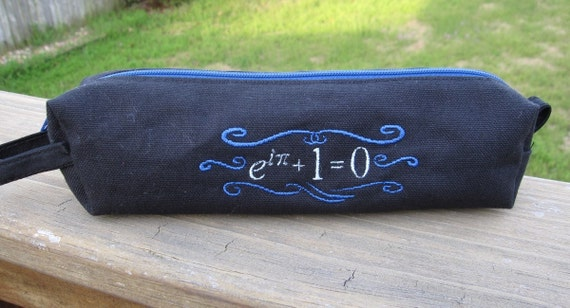Box-and-whisker snowflakes to hang on our Christmas tree: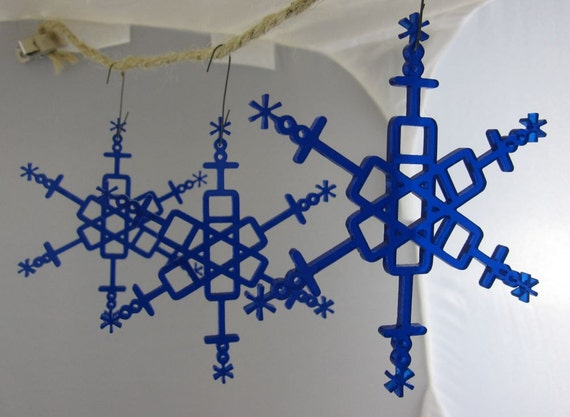And, for me, a wonderfully and subtly punny “Confidence and Power” T-shirt.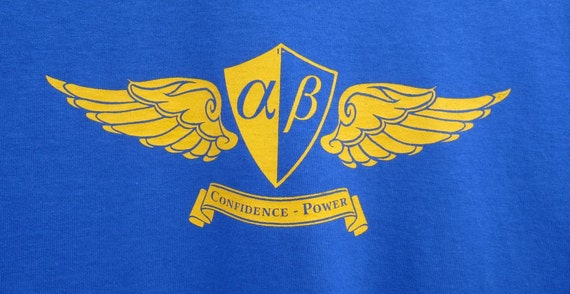Thanks to FiveThirtyEight (see http://fivethirtyeight.com/features/the-fivethirtyeight-2014-holiday-gift-guide/) for pointing me in this direction.For the sake of completeness, here are the math-oriented gifts that I received for Christmas: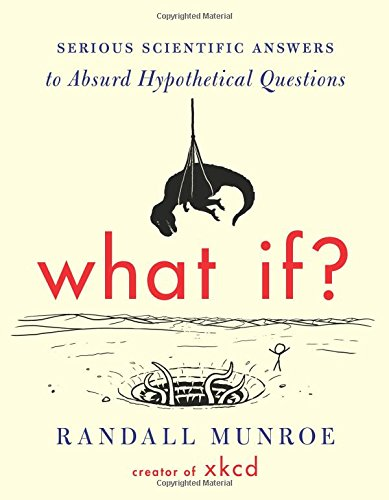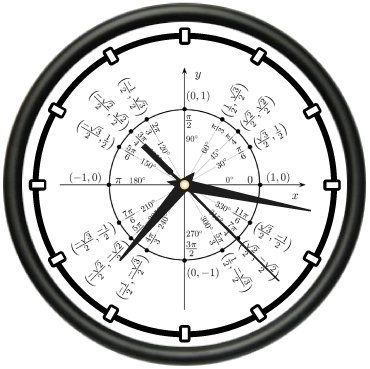# Issues when conducting political polls

The classic application of confidence intervals is political polling: the science of sampling relatively few people to predict the opinions of a large population. However, in the 2010s, the art of political polling — constructing representative samples from a large population — has become more and more difficult.

FiveThirtyEight.com wrote a recent article, Is The Polling Industry in Statis or in Crisis?, about the nuts and bolts of conducting a survey that should provide valuable background information for anyone teaching a course in statistics. From the opening paragraphs:

There is no shortage of reasons to worry about the state of the polling industry. Response rates to political polls are dismal. Even polls that make every effort to contact a representative sample of voters now get no more than 10 percent to complete their surveys — down from about 35 percent in the 1990s.

And there are fewer high-quality polls than there used to be. The cost to commission one can run well into five figures, and it has increased as response rates have declined.1 Under budgetary pressure, many news organizations have understandably preferred to trim their polling budgets rather than lay off newsroom staff.

Cheaper polling alternatives exist, but they come with plenty of problems. “Robopolls,” which use automated scripts rather than live interviewers, often get response rates in the low to mid-single digits. Most are also prohibited by law from calling cell phones, which means huge numbers of people are excluded from their surveys.

How can a poll come close to the outcome when so few people respond to it?

Is The Polling Industry In Stasis Or In Crisis?

# Nuts and Bolts of Political Polls

A standard topic in my statistics class is political polling, which is the canonical example of constructing a confidence interval with a relatively small sample to (hopefully) project the opinions of a large population. Of course, polling is only valid if the sample represents the population at large. This is a natural engagement activity in the fall semester preceding a presidential election.

A recent article on FiveThirtyEight.com, “Are Bad Pollsters Copying Good Pollsters,” does a nice job of explaining some of the nuts and bolts of political polling in an age when selected participants are increasingly unlikely to participate… and also raises the specter of how pollsters using nontraditional methods might consciously or subconconsciously cheating.  A sample (pun intended) from the article:

What’s a nontraditional poll? One that doesn’t abide by the industry’s best practices. So, a survey is nontraditional if it: